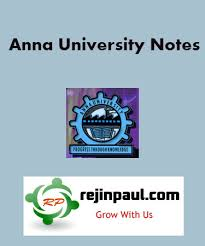### New Update

6/recent/ticker-posts

# GE8292 Engineering Mechanics Syllabus Notes Question Banks with Answers Anna University

## GE8292 Engineering Mechanics Syllabus Notes Question Paper Question Banks Anna University - Regulation 2017 2nd Semester Notes CBCS

Anna University Engineering Mechanics Syllabus Notes Question Bank Question Papers - GE8292 Syllabus Notes
Anna University GE8292 Engineering Mechanics Notes is provided below. GE8292 Notes all 5 units notes are uploaded here. here GE8292 English notes download link is provided and students can download the GE8292 Lecture Notes and can make use of it.GE8292 Engineering Mechanics Syllabus Regulation 2017
UNIT I STATICS OF PARTICLES
Introduction – Units and Dimensions – Laws of Mechanics – Lami’s theorem, Parallelogram and triangular Law of forces – Vectorial representation of forces – Vector operations of forces - additions, subtraction, dot product, cross product – Coplanar Forces – rectangular components – Equilibrium of a particle – Forces in space – Equilibrium of a particle in space – Equivalent systems of forces – Principle of transmissibility .
UNIT II EQUILIBRIUM OF RIGID BODIES
Free body diagram – Types of supports –Action and reaction forces –stable equilibrium – Moments and Couples – Moment of a force about a point and about an axis – Vectorial representation of moments and couples – Scalar components of a moment – Varignon’s theorem – Single equivalent force -Equilibrium of Rigid bodies in two dimensions – Equilibrium of Rigid bodies in three dimensions.
UNIT III PROPERTIES OF SURFACES AND SOLIDS
Centroids and centre of mass – Centroids of lines and areas - Rectangular, circular, triangular areas by integration – T section, I section, - Angle section, Hollow section by using standard formula –Theorems of Pappus - Area moments of inertia of plane areas – Rectangular, circular, triangular areas by integration – T section, I section, Angle section, Hollow section by using standard formula – Parallel axis theorem and perpendicular axis theorem – Principal moments of inertia of plane areas – Principal axes of inertia-Mass moment of inertia –mass moment of inertia for prismatic, cylindrical and spherical solids from first principle – Relation to area moments of inertia.
UNIT IV DYNAMICS OF PARTICLES
Displacements, Velocity and acceleration, their relationship – Relative motion – Curvilinear motion - Newton’s laws of motion – Work Energy Equation– Impulse and Momentum – Impact of elastic bodies.
UNIT V FRICTION AND RIGID BODY DYNAMICS
Friction force – Laws of sliding friction – equilibrium analysis of simple systems with sliding friction wedge friction-. Rolling resistance -Translation and Rotation of Rigid Bodies – Velocity and acceleration – General Plane motion of simple rigid bodies such as cylinder, disc/wheel and sphere.